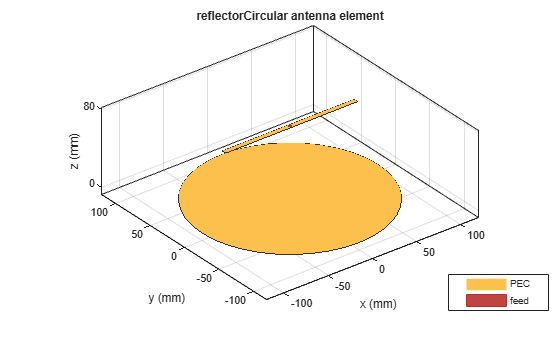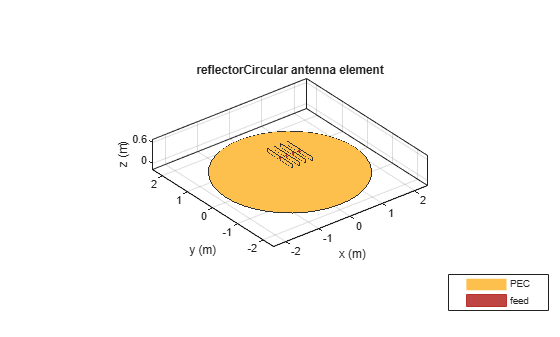# reflectorCircular

Create circular reflector-backed antenna

## Description

Use the `reflectorCircular` object to create a circular reflector-backed antenna. By default the exciter is a dipole. The dimensions are chosen for an operating frequency of 1 GHz.## Creation

### Syntax

``rc = reflectorCircular``
``rc = reflectorCircular(Name,Value)``

### Description

example

````rc = reflectorCircular` creates a circular reflector backed antenna. ```

example

````rc = reflectorCircular(Name,Value)` sets properties using one or more name-value pair. For example, ```rc = reflectorCircular('Radius',0.2)``` creates a circular reflector of radius 0.2 m. Enclose each property name in quotes.```

## Properties

expand all

Antenna type used as an exciter, specified as any single-element antenna object. Except reflector and cavity antenna elements, you can use any of the antenna elements or array elements in the Antenna Toolbox™ as an exciter.

Example: `'Exciter',horn`

Example: `ant.Exciter = horn`

Example: ```ant.Exciter = linearArray('patchMicrostrip')```

Radius of reflector, specified as a scalar in meters.

Example: `'Radius',0.2`

Example: `rc.Radius = 0.2`

Data Types: `double`

Distance between the exciter and the reflector, specified as a scalar in meters.

Example: `'Spacing',7.5e-2`

Example: `rc.Spacing = 7.5e-2`

Data Types: `double`

Type of dielectric material used as a substrate, specified as an object. For more information see, `dielectric`. For more information on dielectric substrate meshing, see Meshing.

Note

The substrate dimensions must be equal to the groundplane dimensions.

Example: ```d = dielectric('FR4'); 'Substrate',d```

Example: ```d = dielectric('FR4'); rc.Substrate = d```

Create probe feed from backing structure to exciter, specified as `0` or `1` or a scalar. By default, probe feed is not enabled.

Example: `'EnableProbeFeed',1`

Example: `rc.EnableProbeFeed = 1`

Data Types: `double` | `logical`

Type of the metal used as a conductor, specified as a metal material object. You can choose any metal from the `MetalCatalog` or specify a metal of your choice. For more information, see `metal`. For more information on metal conductor meshing, see Meshing.

Example: ```m = metal('Copper'); 'Conductor',m```

Example: ```m = metal('Copper'); ant.Conductor = m```

Lumped elements added to the antenna feed, specified as a lumped element object. You can add a load anywhere on the surface of the antenna. By default, the load is at the origin. For more information, see `lumpedElement`.

Example: `'Load',lumpedelement`. `lumpedelement` is the object for the load created using `lumpedElement`.

Example: ```rc.Load = lumpedElement('Impedance',75)```

Tilt angle of the antenna, specified as a scalar or vector with each element unit in degrees. For more information, see Rotate Antennas and Arrays.

Example: `'Tilt',90`

Example: `ant.Tilt = 90`

Example: `'Tilt',[90 90]`,`'TiltAxis',[0 1 0;0 1 1]` tilts the antenna at 90 degrees about the two axes defined by the vectors.

Note

The `wireStack` antenna object only accepts the dot method to change its properties.

Data Types: `double`

Tilt axis of the antenna, specified as:

• Three-element vector of Cartesian coordinates in meters. In this case, each coordinate in the vector starts at the origin and lies along the specified points on the X-, Y-, and Z-axes.

• Two points in space, each specified as three-element vectors of Cartesian coordinates. In this case, the antenna rotates around the line joining the two points in space.

• A string input describing simple rotations around one of the principal axes, 'X', 'Y', or 'Z'.

Example: `'TiltAxis',[0 1 0]`

Example: `'TiltAxis',[0 0 0;0 1 0]`

Example: `ant.TiltAxis = 'Z'`

Note

The `wireStack` antenna object only accepts the dot method to change its properties.

Data Types: `double`

## Object Functions

 `show` Display antenna or array structure; display shape as filled patch `axialRatio` Axial ratio of antenna `beamwidth` Beamwidth of antenna `charge` Charge distribution on metal or dielectric antenna or array surface `current` Current distribution on metal or dielectric antenna or array surface `design` Design prototype antenna or arrays for resonance around specified frequency `efficiency` Radiation efficiency of antenna `EHfields` Electric and magnetic fields of antennas; Embedded electric and magnetic fields of antenna element in arrays `impedance` Input impedance of antenna; scan impedance of array `mesh` Mesh properties of metal or dielectric antenna or array structure `meshconfig` Change mesh mode of antenna structure `optimize` Optimize antenna or array using SADEA optimizer `pattern` Radiation pattern and phase of antenna or array; Embedded pattern of antenna element in array `patternAzimuth` Azimuth pattern of antenna or array `patternElevation` Elevation pattern of antenna or array `rcs` Calculate and plot radar cross section (RCS) of platform, antenna, or array `returnLoss` Return loss of antenna; scan return loss of array `sparameters` Calculate S-parameter for antenna and antenna array objects `vswr` Voltage standing wave ratio of antenna

## Examples

collapse all

Create and view a default circular reflector backed antenna.

`rc = reflectorCircular`
```rc = reflectorCircular with properties: Exciter: [1x1 dipole] Substrate: [1x1 dielectric] GroundPlaneRadius: 0.1000 Spacing: 0.0750 EnableProbeFeed: 0 Conductor: [1x1 metal] Tilt: 0 TiltAxis: [1 0 0] Load: [1x1 lumpedElement] ```
`show(rc)`Create an equiangular spiral backed by a circular reflector.

```ant = reflectorCircular('Exciter',spiralEquiangular,'GroundPlaneRadius', ... 0.02,'Spacing', 0.01); show(ant)```Plot the radiation pattern of the antenna at 4 GHz.

`pattern(ant,4e9)`Create a linear array of the dipole antennas.

```d = dipole('Length',1); la = linearArray('Element',d,'NumElements',4,'ElementSpacing',0.2,'Tilt',90);```

Create a linear array with circular reflector backing structure.

`ant = reflectorCircular('Exciter',la,'GroundPlaneRadius',2,'Spacing',0.5)`
```ant = reflectorCircular with properties: Exciter: [1x1 linearArray] Substrate: [1x1 dielectric] GroundPlaneRadius: 2 Spacing: 0.5000 EnableProbeFeed: 0 Conductor: [1x1 metal] Tilt: 0 TiltAxis: [1 0 0] Load: [1x1 lumpedElement] ```
`show(ant)`Create a circular reflector-backed circular array of cylindrical DRAs.

```e = draCylindrical; ca = circularArray('Element',e,'Radius',0.05); ant = reflectorCircular('Exciter',ca,'GroundPlaneRadius',0.2)```
```ant = reflectorCircular with properties: Exciter: [1x1 circularArray] Substrate: [1x1 dielectric] GroundPlaneRadius: 0.2000 Spacing: 0.0750 EnableProbeFeed: 0 Conductor: [1x1 metal] Tilt: 0 TiltAxis: [1 0 0] Load: [1x1 lumpedElement] ```
`show(ant)`